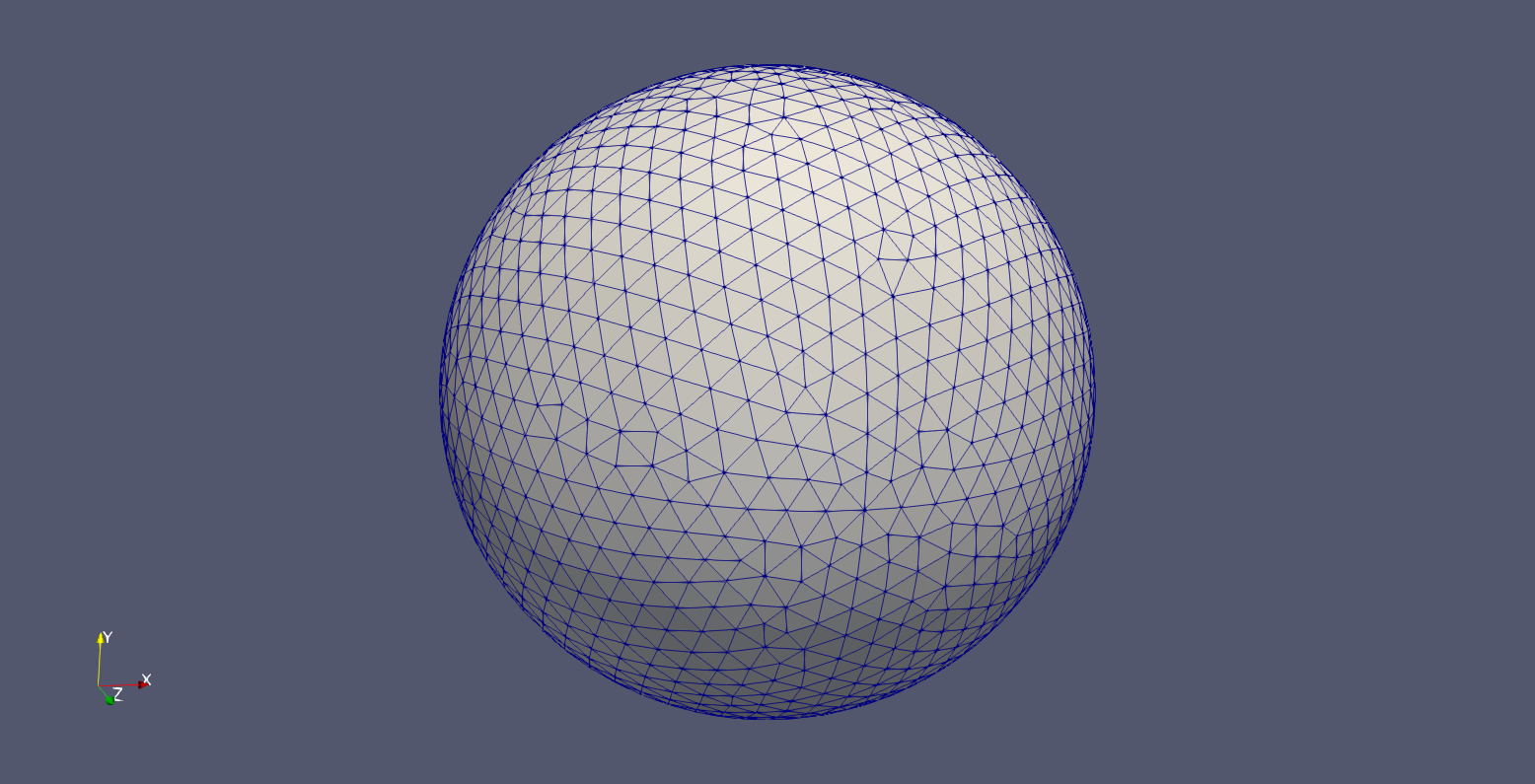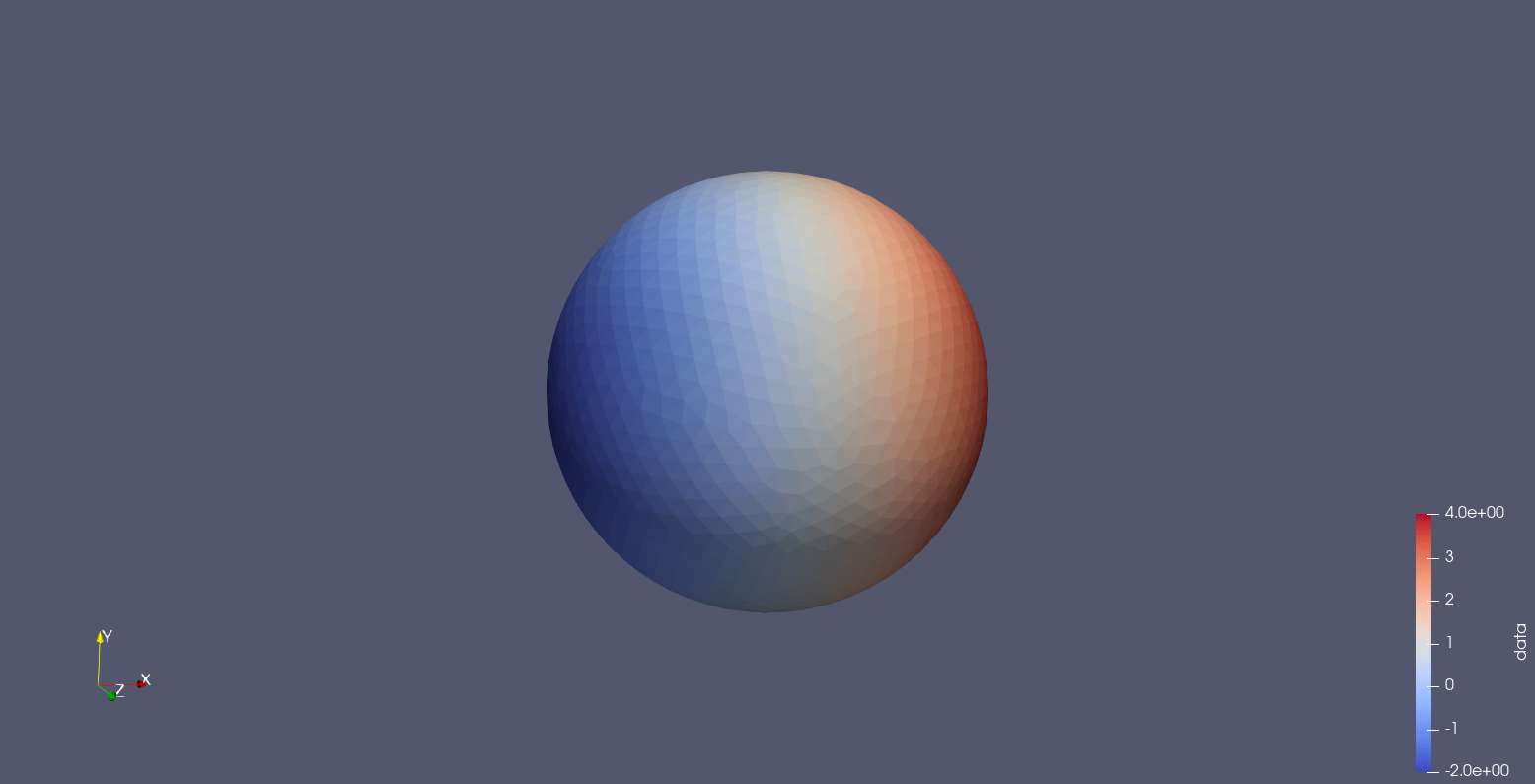# Solving your first problem with Bempp

Before following this tutorial, you will need to install Bempp. Once you have Bempp installed, open a Jupyter notebook or a Python or IPython terminal and you are ready to begin.

### Importing Bempp

First, you need to import Bempp.

Bempp is split into two parts: bempp.api and bempp.core: bempp.api contains the library’s Python functionality and bempp.core contains the interfaces to the fast OpenCL (or C++ if you’re using the previous version) computational kernel. As a user you (almost certainly) want to begin by importing bempp.api:

import bempp.api


### Generating a grid

Bempp solves discretised problems on grids (or meshes) of triangular cells. Before solving your problem, you must create or import your grid.

In this example, we will use a sphere. The following code snippet will create your grid.

grid = bempp.api.shapes.sphere(h=0.1)
grid.plot()


If you are using a Jupyter notebook, then the second line in this snippet will visualise your grid using plotly. If you are not using a notebook, you will need to change the plotting backend in order to visualise your grid. The following code, for example, will visualise your grid using Paraview.

bempp.api.PLOT_BACKEND = "paraview"
grid.plot()


If all is well, you will now have created and visulaised your grid.### Defining a function space

Next, you need to build a function space on the grid. In this case, we use a space of piecewise constant polynomials, or order 0 discontinuous polynomials (DP).

The following code snippet will create this function space.

space = bempp.api.function_space(grid, "DP", 0)


### Building boundary operators

Next, you can build boundary operators using the spaces you have defined. In this example, we construct a Laplace single layer boundary operator.

This operator can be defined using the following Python snippet.

slp = bempp.api.operators.boundary.laplace.single_layer(space, space, space)


### Constructing a grid function

Next, we construct a grid function containing your right-hand-side data. In this example, we use a Python function to construct our grid function. As our function is real-valued, we must use the @bempp.api.real_callable decorator so that Bempp can use the correct number types in its compiled code.

@bempp.api.real_callable
def f(x, n, domain_index, result):
result = x + 1

rhs = bempp.api.GridFunction(space, fun=f)


### Solving

Now that you have constructed all the necessary objects, you are ready to solve the discretised problem. In this case, we use GMRES to solve the problem.

sol, info = bempp.api.linalg.gmres(slp, rhs)


Finally, you can visualise your solution:

sol.plot()### What’s next?

A list of further documentation and tutorials can be found on the documentation page.